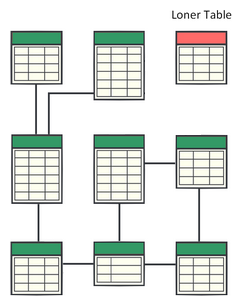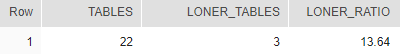## Loner Ratio - how many tables have no relationships in Snowflake

Article for:

Query below returns number of tables that have no foreign key constraint and are not referenced by any foreign key either. Tables like this we call Loner Tables.## Query

``````select count(distinct tab.table_schema ||'.'|| tab.table_name) as tables,
count(distinct tab.table_schema ||'.'|| tab.table_name)
- count(distinct rel.table_schema ||'.'|| rel.table_name)
as loner_tables,
round(100.0*(count(distinct tab.table_schema ||'.'||  tab.table_name)
- count(distinct rel.table_schema ||'.'||  rel.table_name))
/count(distinct tab.table_schema ||'.'||  tab.table_name),2)
as loner_ratio
from information_schema.tables tab
left join (
select distinct tco.table_schema ,tco.table_name
from information_schema.referential_constraints rco
join information_schema.table_constraints tco
on rco.unique_constraint_name = tco.constraint_name
and rco.unique_constraint_schema = tco.table_schema
union all
select distinct tco.table_schema, tco.table_name
from information_schema.referential_constraints rco
join information_schema.table_constraints tco
on rco.constraint_name = tco.constraint_name
and rco.constraint_schema = tco.table_schema
) rel on rel.table_name = tab.table_name
and rel.table_schema = tab.table_schema
where tab.table_schema != 'INFORMATION_SCHEMA'
and tab.table_type ='BASE TABLE';
``````

## Columns

• tables - number of all tables in database
• loner_tables - number of Loner Tables in the database
• loner_ratio - Loner Ratio - % of Loner Tables in the database

## Rows

• Scope of rows: query returns one row

## Sample results

Loner Ratio in our sample database is 13.64%, meaning that 13.64% of tables are not related to other tables.0